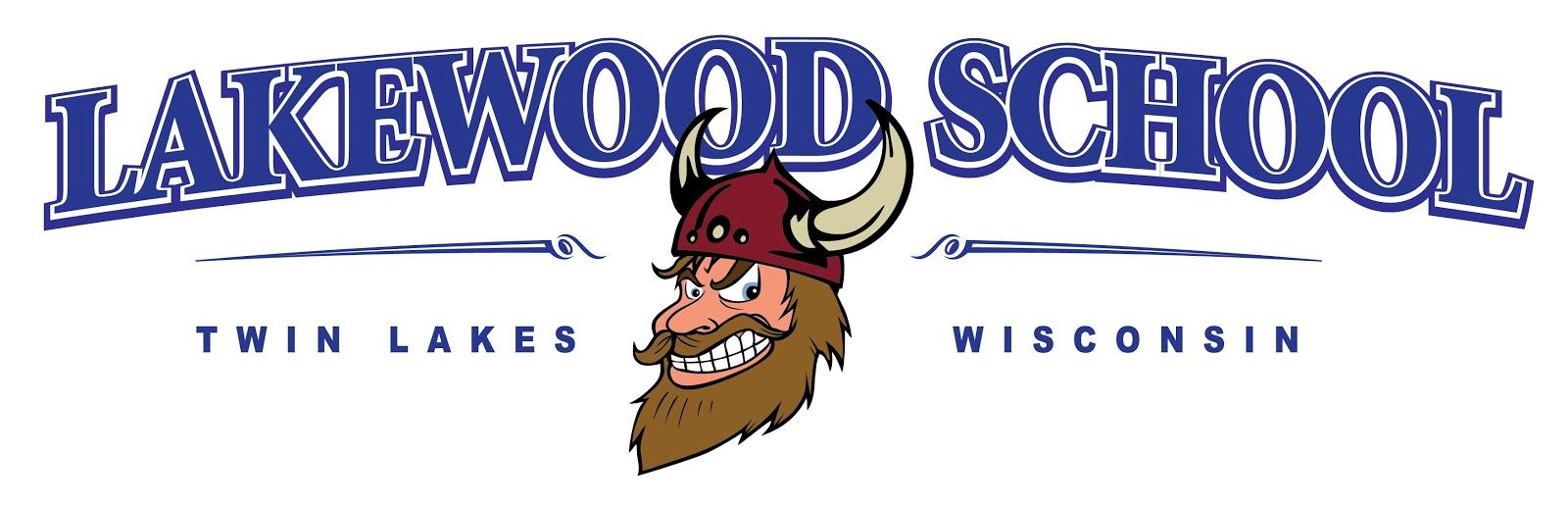• Select a School
• LanguageFourth Grade Curriculum Printable ELA English, Language Arts I can read unfamiliar multisyllabic words. I can read on-level text for understanding. I can read on level text, including poetry, fluently. I can use context to help me read and self-correct if necessary. I refer back to the text when explaining. I can determine a theme and summarize a text. I can use details in the text to describe a character, setting or event. I can compare and contrast point of view in different stories. I can compare and contrast themes, topics and patterns of events in stories from different cultures. I can refer to the text when explaining and inferring. I can summarize text. I can describe the structure of a text. I can compare and contrast two different perspectives of the same topic. I can explain how information presented is needed to understand the text. I can explain how an author supports his points in a text. I can state my opinion, group related ideas and provide reasons to support my facts using transitional phrases. I can write about a topic using topic specific vocabulary, specific examples from outside sources, paragraphs and text features to aid in comprehension. I can write a narrative using transitional words and sensory details. MATH Operations and Algebraic Thinking, Numbers and Operations in Base Ten, Measurement and Data, Geometry Solve multistep word problems posed with whole numbers and having whole-number answers using the four operations, including problems in which remainders must be interpreted. Represent these problems using equations with a letter standing for the unknown quantity. Assess the reasonableness of answers using mental computation and estimation strategies including rounding. Find all factor pairs for a whole number in the range 1-100. Recognize that a whole number is a multiple of each of its factors. Determine whether a given whole number in the range 1-100 is a multiple of a given one-digit number.  Determine whether a given whole number in the range 1-100 is prime or composite. Read and write multi-digit whole numbers using base-ten numerals, number names, and expanded form. Compare two multi-digit numbers based on meanings of the digits in each place, using >, =, and < symbols to record the results of comparisons. Use place value understanding to round multi-digit whole numbers to any place. Fluently add and subtract multi-digit whole numbers using the standard algorithm. Find whole-number quotients and remainders with up to four-digit dividends and one-digit divisors, using strategies based on place value, the properties of operations, and/or the relationship between multiplication and division. Illustrate and explain the calculation by using equations, rectangular arrays, and/or area models. Know relative sizes of measurement units within one system of units including km, m, cm; kg, g; lb, oz.; l, ml; hr, min, sec. Within a single system of measurement, express measurements in a larger unit in terms of a smaller unit. Record measurement equivalents in a two-column table. For example, know that 1 ft is 12 times as long as 1 in. Express the length of a 4 ft snake as 48 in. Generate a conversion table for feet and inches listing the number pairs (1, 12), (2, 24), (3, 36) Apply the area and perimeter formulas for rectangles in real world and mathematical problems. Recognize angles as geometric shapes that are formed wherever two rays share a common endpoint, and understand concepts of angle measurement. Measure angles in whole-number degrees using a protractor. Sketch angles of specified measure. Recognize angle measure as additive. When an angle is decomposed into non-overlapping parts, the angle measure of the whole is the sum of the angle measures of the parts. Solve addition and subtraction problems to find unknown angles on a diagram in real world and mathematical problems. Explain why a fraction a/b is equivalent to a fraction (n × a)/(n × b) by using visual fraction models, with attention to how the number and size of the parts differ even though the two fractions themselves are the same size. Use this principle to recognize and generate equivalent fractions. Compare two fractions with different numerators and different denominators, e.g., by creating common denominators or numerators, or by comparing to a benchmark fraction such as 1/2. Recognize that comparisons are valid only when the two fractions refer to the same whole. Record the results of comparisons with symbols >, =, or <, and justify the conclusions. Use decimal notation for fractions with denominators 10 or 100. Draw points, lines, line segments, rays, angles (right, acute, obtuse), and perpendicular and parallel lines. Identify these in two-dimensional figures. Classify two-dimensional figures based on the presence or absence of parallel or perpendicular lines, or the presence or absence of angles of a specified size. Recognize right triangles as a category, and identify right triangles. Recognize a line of symmetry for a two-dimensional figure as a line across the figure such that the figure can be folded along the line into matching parts. Identify line-symmetric figures and draw lines of symmetry.